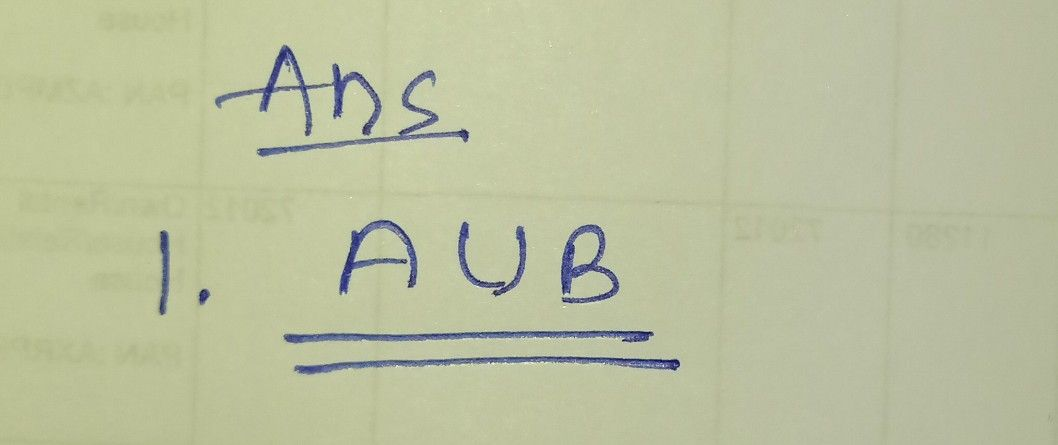Symbol
ProblemActivity $y1$ $Di\pi ecbons$ Identify the set notation based on the shaded region of the Venn diagram. Write your answer on the space provided. $A$ $B$ $ExamD|e$ Set $notaion$ B' $A$ $B$ $1\right)$ $B$
7th-9th grade
Other
Search count: 107
SolutionQanda teacher - Ishita